snssde1d()

Assume that we want to describe the following SDE:

It? form3:

$\begin{equation}\label{eq:05} dX_{t} = \frac{1}{2}\theta^{2} X_{t} dt + \theta X_{t} dW_{t},\qquad X_{0}=x_{0} > 0 \end{equation}$

Stratonovich form: $\begin{equation}\label{eq:06} dX_{t} = \frac{1}{2}\theta^{2} X_{t} dt +\theta X_{t} \circ dW_{t},\qquad X_{0}=x_{0} > 0 \end{equation}$

In the above $$f(t,x)=\frac{1}{2}\theta^{2} x$$ and $$g(t,x)= \theta x$$ ($$\theta > 0$$), $$W_{t}$$ is a standard Wiener process. To simulate this models using snssde1d() function we need to specify:

• The drift and diffusion coefficients as R expressions that depend on the state variable x and time variable t.
• The number of simulation steps N=1000 (by default: N=1000).
• The number of the solution trajectories to be simulated by M=1000 (by default: M=1).
• The initial conditions t0=0, x0=10 and end time T=1 (by default: t0=0, x0=0 and T=1).
• The integration step size Dt=0.001 (by default: Dt=(T-t0)/N).
• The choice of process types by the argument type="ito" for Ito or type="str" for Stratonovich (by default type="ito").
• The numerical method to be used by method (by default method="euler").
R> theta = 0.5
R> f <- expression( (0.5*theta^2*x) )
R> g <- expression( theta*x )
R> mod1 <- snssde1d(drift=f,diffusion=g,x0=10,M=1000,type="ito") # Using It?
R> mod2 <- snssde1d(drift=f,diffusion=g,x0=10,M=1000,type="str") # Using Stratonovich
R> mod1
Itô Sde 1D:
| dX(t) = (0.5 * theta^2 * X(t)) * dt + theta * X(t) * dW(t)
Method:
| Euler scheme with order 0.5
Summary:
| Size of process   | N  = 1001.
| Number of simulation  | M  = 1000.
| Initial value     | x0 = 10.
| Time of process   | t in [0,1].
| Discretization    | Dt = 0.001.
R> mod2
Stratonovich Sde 1D:
| dX(t) = (0.5 * theta^2 * X(t)) * dt + theta * X(t) o dW(t)
Method:
| Euler scheme with order 0.5
Summary:
| Size of process   | N  = 1001.
| Number of simulation  | M  = 1000.
| Initial value     | x0 = 10.
| Time of process   | t in [0,1].
| Discretization    | Dt = 0.001.

Using Monte-Carlo simulations, the following statistical measures (S3 method) for class snssde1d() can be approximated for the $$X_{t}$$ process at any time $$t$$:

• The expected value $$\text{E}(X_{t})$$ at time $$t$$, using the command mean.
• The variance $$\text{Var}(X_{t})$$ at time $$t$$, using the command moment with order=2 and center=TRUE.
• The median $$\text{Med}(X_{t})$$ at time $$t$$, using the command Median.
• The mode $$\text{Mod}(X_{t})$$ at time $$t$$, using the command Mode.
• The quartile of $$X_{t}$$ at time $$t$$, using the command quantile.
• The maximum and minimum of $$X_{t}$$ at time $$t$$, using the command min and max.
• The skewness and the kurtosis of $$X_{t}$$ at time $$t$$, using the command skewness and kurtosis.
• The coefficient of variation (relative variability) of $$X_{t}$$ at time $$t$$, using the command cv.
• The central moments up to order $$p$$ of $$X_{t}$$ at time $$t$$, using the command moment.
• The empirical $$\alpha \%$$ confidence interval of expected value $$\text{E}(X_{t})$$ at time $$t$$ (from the $$2.5th$$ to the $$97.5th$$ percentile), using the command bconfint.
• The result summaries of the results of Monte-Carlo simulation at time $$t$$, using the command summary.

The following statistical measures (S3 method) for class snssde1d() can be approximated for the $$X_{t}$$ process at any time $$t$$, for example at=1:

R> s = 1
R> mean(mod1, at = s)
 11.141
R> moment(mod1, at = s , center = TRUE , order = 2) ## variance
 31.678
R> Median(mod1, at = s)
 9.9339
R> Mode(mod1, at =s)
 7.8949
R> quantile(mod1 , at = s)
0%     25%     50%     75%    100%
1.7029  6.9402  9.9339 14.1501 40.1214
R> kurtosis(mod1 , at = s)
 5.049
R> skewness(mod1 , at = s)
 1.2197
R> cv(mod1 , at = s )
 0.50546
R> min(mod1 , at = s)
 1.7029
R> max(mod1 , at = s)
 40.121
R> moment(mod1, at = s , center= TRUE , order = 4)
 5076.7
R> moment(mod1, at = s , center= FALSE , order = 4)
 53776

The summary of the results of mod1 and mod2 at time $$t=1$$ of class snssde1d() is given by:

R> summary(mod1, at = 1)

Monte-Carlo Statistics for X(t) at time t = 1

Mean                  11.14059
Variance              31.70956
Median                 9.93394
Mode                   7.89493
First quartile         6.94024
Third quartile        14.15014
Minimum                1.70286
Maximum               40.12143
Skewness               1.21974
Kurtosis               5.04898
Coef-variation         0.50546
3th-order moment     217.79819
4th-order moment    5076.73338
5th-order moment   88870.00801
6th-order moment 1991341.09731
R> summary(mod2, at = 1)

Monte-Carlo Statistics for X(t) at time t = 1

Mean                   9.79629
Variance              30.56349
Median                 8.62333
Mode                   6.37665
First quartile         5.98119
Third quartile        11.98768
Minimum                1.86562
Maximum               41.72127
Skewness               1.78504
Kurtosis               7.96468
Coef-variation         0.56434
3th-order moment     301.61461
4th-order moment    7440.02410
5th-order moment  171076.23474
6th-order moment 4520033.64638

Hence we can just make use of the rsde1d() function to build our random number generator for the conditional density of the $$X_{t}|X_{0}$$ ($$X_{t}^{\text{mod1}}| X_{0}$$ and $$X_{t}^{\text{mod2}}|X_{0}$$) at time $$t = 1$$.

R> x1 <- rsde1d(object = mod1, at = 1)  # X(t=1) | X(0)=x0 (It? SDE)
R> x2 <- rsde1d(object = mod2, at = 1)  # X(t=1) | X(0)=x0 (Stratonovich SDE)
  7.8535  9.7246 21.2346  8.3660 12.9236 14.8307  9.7619 12.7098
 17.6658 14.5872
  7.9676 10.0006  9.8624  5.4222  9.1462  8.5774 19.4367 10.6745
  7.3757 15.1997
R> summary(data.frame(x1,x2))
x1              x2
Min.   : 1.70   Min.   : 1.87
1st Qu.: 6.94   1st Qu.: 5.98
Median : 9.93   Median : 8.62
Mean   :11.14   Mean   : 9.80
3rd Qu.:14.15   3rd Qu.:11.99
Max.   :40.12   Max.   :41.72

The function dsde1d() can be used to show the Approximate transitional density for $$X_{t}|X_{0}$$ at time $$t-s=1$$ with log-normal curves:

R> mu1 = log(10); sigma1= sqrt(theta^2)  # log mean and log variance for mod1
R> mu2 = log(10)-0.5*theta^2 ; sigma2 = sqrt(theta^2) # log mean and log variance for mod2
R> AppdensI <- dsde1d(mod1, at = 1)
R> AppdensS <- dsde1d(mod2, at = 1)
R> plot(AppdensI , dens = function(x) dlnorm(x,meanlog=mu1,sdlog = sigma1))
R> plot(AppdensS , dens = function(x) dlnorm(x,meanlog=mu2,sdlog = sigma2))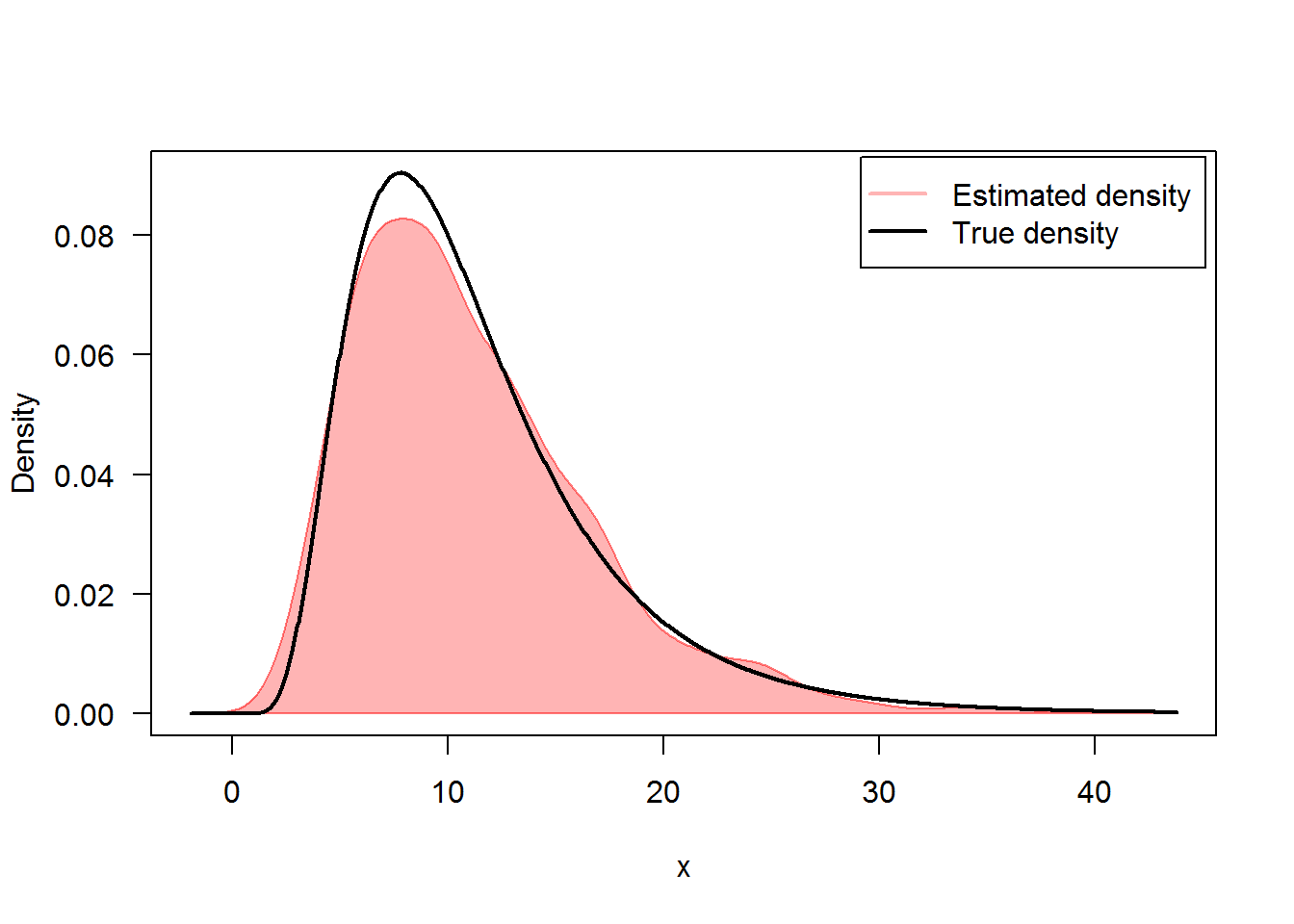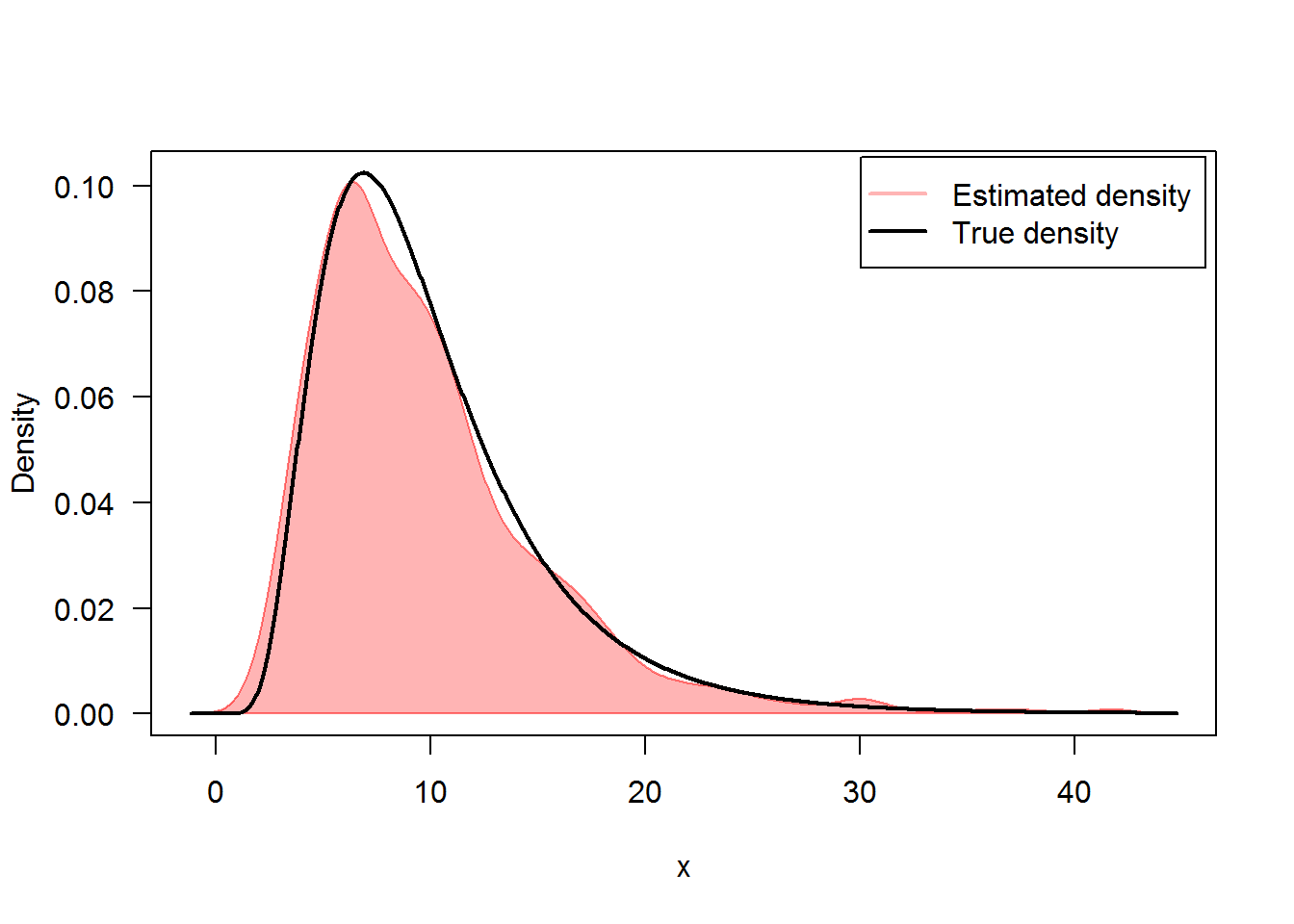In Figure 2, we present the flow of trajectories, the mean path (red lines) of solution of and , with their empirical $$95\%$$ confidence bands, that is to say from the $$2.5th$$ to the $$97.5th$$ percentile for each observation at time $$t$$ (blue lines):

R> ## It?
R> plot(mod1,ylab=expression(X^mod1))
R> lines(time(mod1),apply(mod1$X,1,mean),col=2,lwd=2) R> lines(time(mod1),apply(mod1$X,1,bconfint,level=0.95)[1,],col=4,lwd=2)
R> lines(time(mod1),apply(mod1$X,1,bconfint,level=0.95)[2,],col=4,lwd=2) R> legend("topleft",c("mean path",paste("bound of", 95,"% confidence")),inset = .01,col=c(2,4),lwd=2,cex=0.8) R> ## Stratonovich R> plot(mod2,ylab=expression(X^mod2)) R> lines(time(mod2),apply(mod2$X,1,mean),col=2,lwd=2)
R> lines(time(mod2),apply(mod2$X,1,bconfint,level=0.95)[1,],col=4,lwd=2) R> lines(time(mod2),apply(mod2$X,1,bconfint,level=0.95)[2,],col=4,lwd=2)
R> legend("topleft",c("mean path",paste("bound of",95,"% confidence")),col=c(2,4),inset =.01,lwd=2,cex=0.8)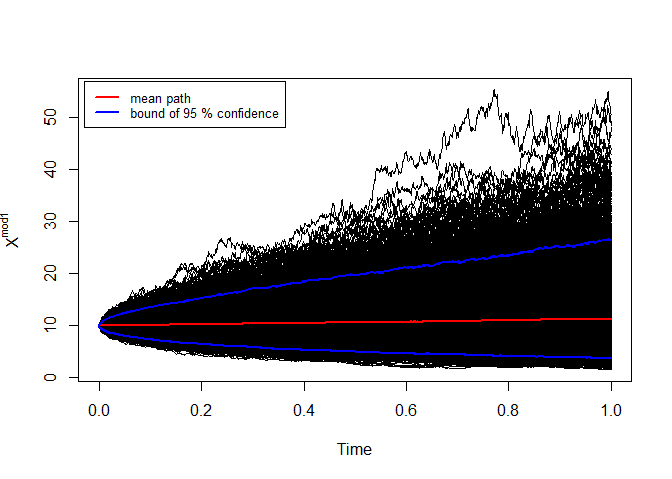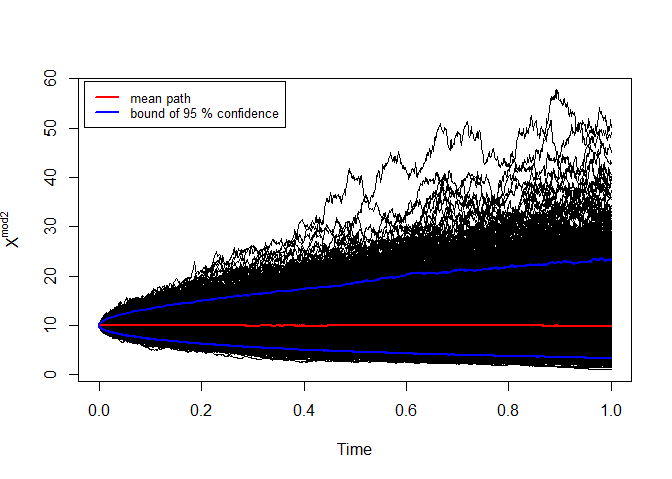mod1: It? and mod2: Stratonovich

snssde2d()

The following $$2$$-dimensional SDE’s with a vector of drift and a diagonal matrix of diffusion coefficients:

It? form: $\begin{equation}\label{eq:09} \begin{cases} dX_t = f_{x}(t,X_{t},Y_{t}) dt + g_{x}(t,X_{t},Y_{t}) dW_{1,t}\\ dY_t = f_{y}(t,X_{t},Y_{t}) dt + g_{y}(t,X_{t},Y_{t}) dW_{2,t} \end{cases} \end{equation}$

Stratonovich form: $\begin{equation}\label{eq:10} \begin{cases} dX_t = f_{x}(t,X_{t},Y_{t}) dt + g_{x}(t,X_{t},Y_{t}) \circ dW_{1,t}\\ dY_t = f_{y}(t,X_{t},Y_{t}) dt + g_{y}(t,X_{t},Y_{t}) \circ dW_{2,t} \end{cases} \end{equation}$ $$W_{1,t}$$ and $$W_{2,t}$$ is a two independent standard Wiener process. To simulate $$2d$$ models using snssde2d() function we need to specify:

• The drift (2d) and diffusion (2d) coefficients as R expressions that depend on the state variable x, y and time variable t.
• The number of simulation steps N (default: N=1000).
• The number of the solution trajectories to be simulated by M (default: M=1).
• The initial conditions t0, x0 and end time T (default: t0=0, x0=c(0,0) and T=1).
• The integration step size Dt (default: Dt=(T-t0)/N).
• The choice of process types by the argument type="ito" for Ito or type="str" for Stratonovich (default type="ito").
• The numerical method to be used by method (default method="euler").

Ornstein-Uhlenbeck process and its integral

The Ornstein-Uhlenbeck (OU) process has a long history in physics. Introduced in essence by Langevin in his famous 1908 paper on Brownian motion, the process received a more thorough mathematical examination several decades later by Uhlenbeck and Ornstein (1930). The OU process is understood here to be the univariate continuous Markov process $$X_t$$. In mathematical terms, the equation is written as an Ito equation: $\begin{equation}\label{eq016} dX_t = -\frac{1}{\mu} X_t dt + \sqrt{\sigma} dW_t,\quad X_{0}=x_{0} \end{equation}$ In these equations, $$\mu$$ and $$\sigma$$ are positive constants called, respectively, the relaxation time and the diffusion constant. The time integral of the OU process $$X_t$$ (or indeed of any process $$X_t$$) is defined to be the process $$Y_t$$ that satisfies: $\begin{equation}\label{eq017} Y_{t} = Y_{0}+\int X_{t} dt \Leftrightarrow dY_t = X_{t} dt ,\quad Y_{0}=y_{0} \end{equation}$ $$Y_t$$ is not itself a Markov process; however, $$X_t$$ and $$Y_t$$ together comprise a bivariate continuous Markov process. We wish to find the solutions $$X_t$$ and $$Y_t$$ to the coupled time-evolution equations: $\begin{equation}\label{eq018} \begin{cases} dX_t = -\frac{1}{\mu} X_t dt + \sqrt{\sigma} dW_t\\ dY_t = X_{t} dt \end{cases} \end{equation}$

We simulate a flow of $$1000$$ trajectories of $$(X_{t},Y_{t})$$, with integration step size $$\Delta t = 0.01$$, and using second Milstein method.

Itô Sde 2D:
| dX(t) = -(X(t)/mu) * dt + sqrt(sigma) * dW1(t)
| dY(t) = X(t) * dt + 0 * dW2(t)
Method:
| Second-order Milstein scheme
Summary:
| Size of process   | N  = 1001.
| Number of simulation  | M  = 1000.
| Initial values    | (x0,y0) = (5,0).
| Time of process   | t in [0,10].
| Discretization    | Dt = 0.01.

The following statistical measures (S3 method) for class snssde2d() can be approximated for the $$(X_{t},Y_{t})$$ process at any time $$t$$, for example at=5:

  0.92679 12.21988
 0.68889 6.66566
  0.93172 12.17180
  1.0114 12.2733
$x 0% 25% 50% 75% 100% -1.76975 0.32673 0.93172 1.47083 3.93978$y
0%     25%     50%     75%    100%
1.1376 10.4418 12.1718 13.9680 20.7411
 3.0766 3.2350
 0.040533 0.055974
 0.89601 0.21138
 -1.7698  1.1376
  3.9398 20.7411
   1.463 144.020
     5.837 28461.365

The summary of the results of mod2d at time $$t=5$$ of class snssde2d() is given by:

Monte-Carlo Statistics for (X(t),Y(t)) at time t = 5
X          Y
Mean              0.92679   12.21988
Variance          0.68958    6.67233
Median            0.93172   12.17180
Mode              1.01144   12.27334
First quartile    0.32673   10.44181
Third quartile    1.47083   13.96801
Minimum          -1.76975    1.13758
Maximum           3.93978   20.74114
Skewness          0.04053    0.05597
Kurtosis          3.07656    3.23495
Coef-variation    0.89601    0.21138
3th-order moment  0.02321    0.96472
4th-order moment  1.46296  144.02011
5th-order moment  0.09333  -55.09065
6th-order moment  5.28267 6095.24983

For plotting (back in time) using the command plot, the results of the simulation are shown in Figure 3.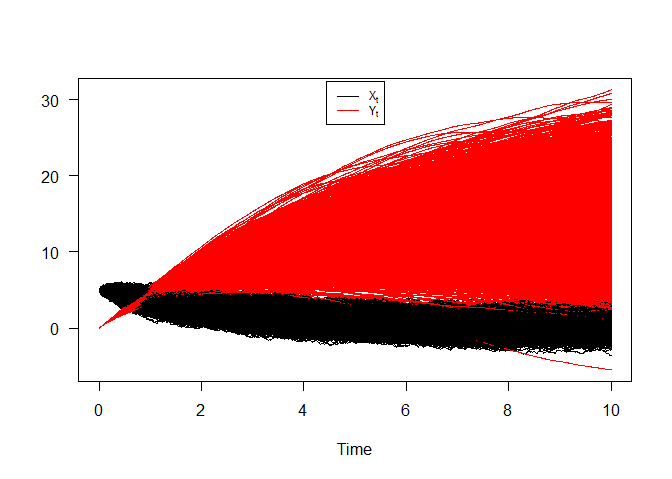Ornstein-Uhlenbeck process and its integral

Take note of the well known result, which can be derived from either this equations. That for any $$t > 0$$ the OU process $$X_t$$ and its integral $$Y_t$$ will be the normal distribution with mean and variance given by: $\begin{cases} \text{E}(X_{t}) =x_{0} e^{-t/\mu} &\text{and}\quad\text{Var}(X_{t})=\frac{\sigma \mu}{2} \left (1-e^{-2t/\mu}\right )\\ \text{E}(Y_{t}) = y_{0}+x_{0}\mu \left (1-e^{-t/\mu}\right ) &\text{and}\quad\text{Var}(Y_{t})=\sigma\mu^{3}\left (\frac{t}{\mu}-2\left (1-e^{-t/\mu}\right )+\frac{1}{2}\left (1-e^{-2t/\mu}\right )\right ) \end{cases}$

Hence we can just make use of the rsde2d() function to build our random number for $$(X_{t},Y_{t})$$ at time $$t = 10$$.

x       y
1  -0.40797 20.2867
2  -0.19133 12.1643
3  -0.75371  9.4854
4   0.30984 13.3197
5  -0.87097 13.6577
6   1.29402 16.1173
7   0.63369 13.9212
8   1.37924 18.2539
9  -0.44067 20.0510
10 -1.38257  3.5238
x                y
Min.   :-3.102   Min.   :-2.02
1st Qu.:-0.488   1st Qu.:11.03
Median : 0.168   Median :13.91
Mean   : 0.151   Mean   :14.27
3rd Qu.: 0.765   3rd Qu.:17.75
Max.   : 2.860   Max.   :33.57
x       y
x 0.8335  2.2577
y 2.2577 25.4355

Figure 4, show simulation results for moments of system :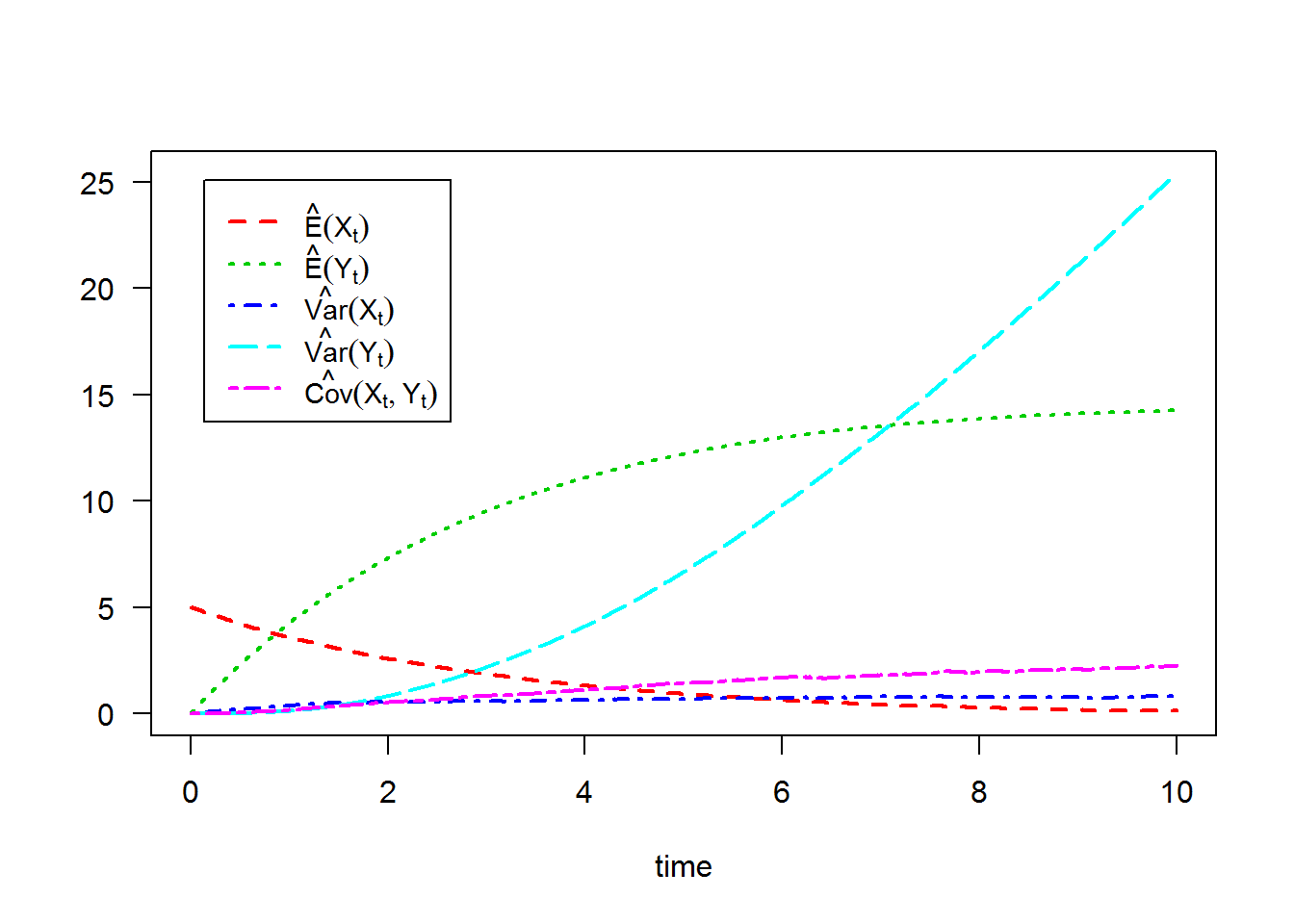For each SDE type and for each numerical scheme, the density of $$X_t$$ and $$Y_t$$ at time $$t=10$$ are reported using dsde2d() function, see e.g. Figure 5: the marginal density of $$X_t$$ and $$Y_t$$ at time $$t=10$$.

Marginal density of X(t-t0)|X(t0)=5 at time t = 10

Data: x (1000 obs.);    Bandwidth 'bw' = 0.2064

x                f(x)
Min.   :-3.7208   Min.   :0.00002
1st Qu.:-1.9207   1st Qu.:0.00727
Median :-0.1207   Median :0.06398
Mean   :-0.1207   Mean   :0.13875
3rd Qu.: 1.6794   3rd Qu.:0.27940
Max.   : 3.4794   Max.   :0.42392

Marginal density of Y(t-t0)|Y(t0)=0 at time t = 10

Data: y (1000 obs.);    Bandwidth 'bw' = 1.133

y               f(y)
Min.   :-5.422   Min.   :0.000004
1st Qu.: 5.177   1st Qu.:0.000781
Median :15.776   Median :0.008865
Mean   :15.776   Mean   :0.023565
3rd Qu.:26.375   3rd Qu.:0.046913
Max.   :36.973   Max.   :0.084134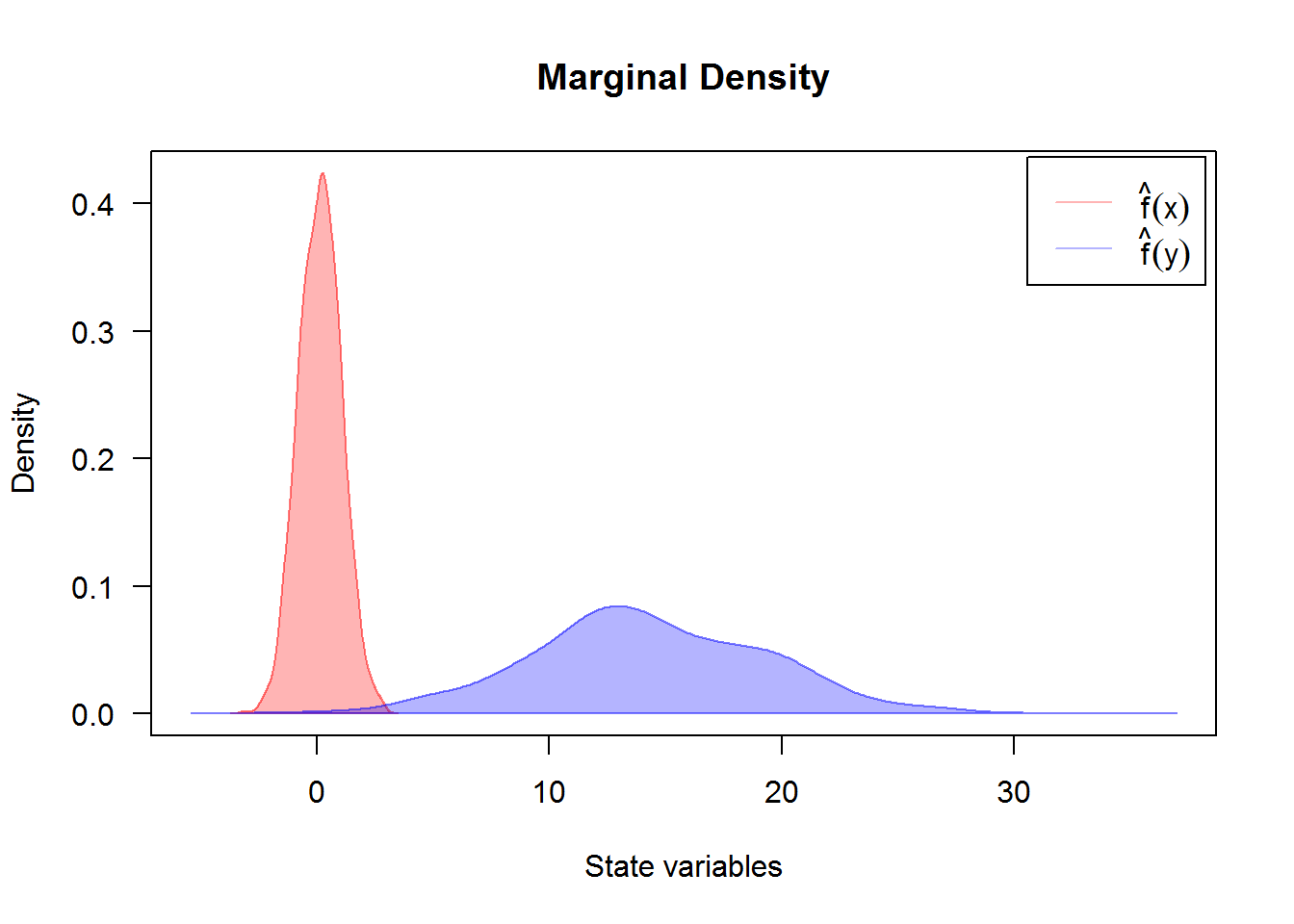Created using dsde2d() plotted in (x, y)-space with dim = 2. A contour and image plot of density obtained from a realization of system $$(X_{t},Y_{t})$$ at time t=10.

Joint density of (X(t-t0),Y(t-t0)|X(t0)=5,Y(t0)=0) at time t = 10

Data: (x,y) (2 x 1000 obs.);

x                  y              f(x,y)
Min.   :-3.10162   Min.   :-2.022   Min.   :0.000000
1st Qu.:-1.61115   1st Qu.: 6.877   1st Qu.:0.000028
Median :-0.12068   Median :15.776   Median :0.000690
Mean   :-0.12068   Mean   :15.776   Mean   :0.004603
3rd Qu.: 1.36980   3rd Qu.:24.675   3rd Qu.:0.004779
Max.   : 2.86027   Max.   :33.574   Max.   :0.037396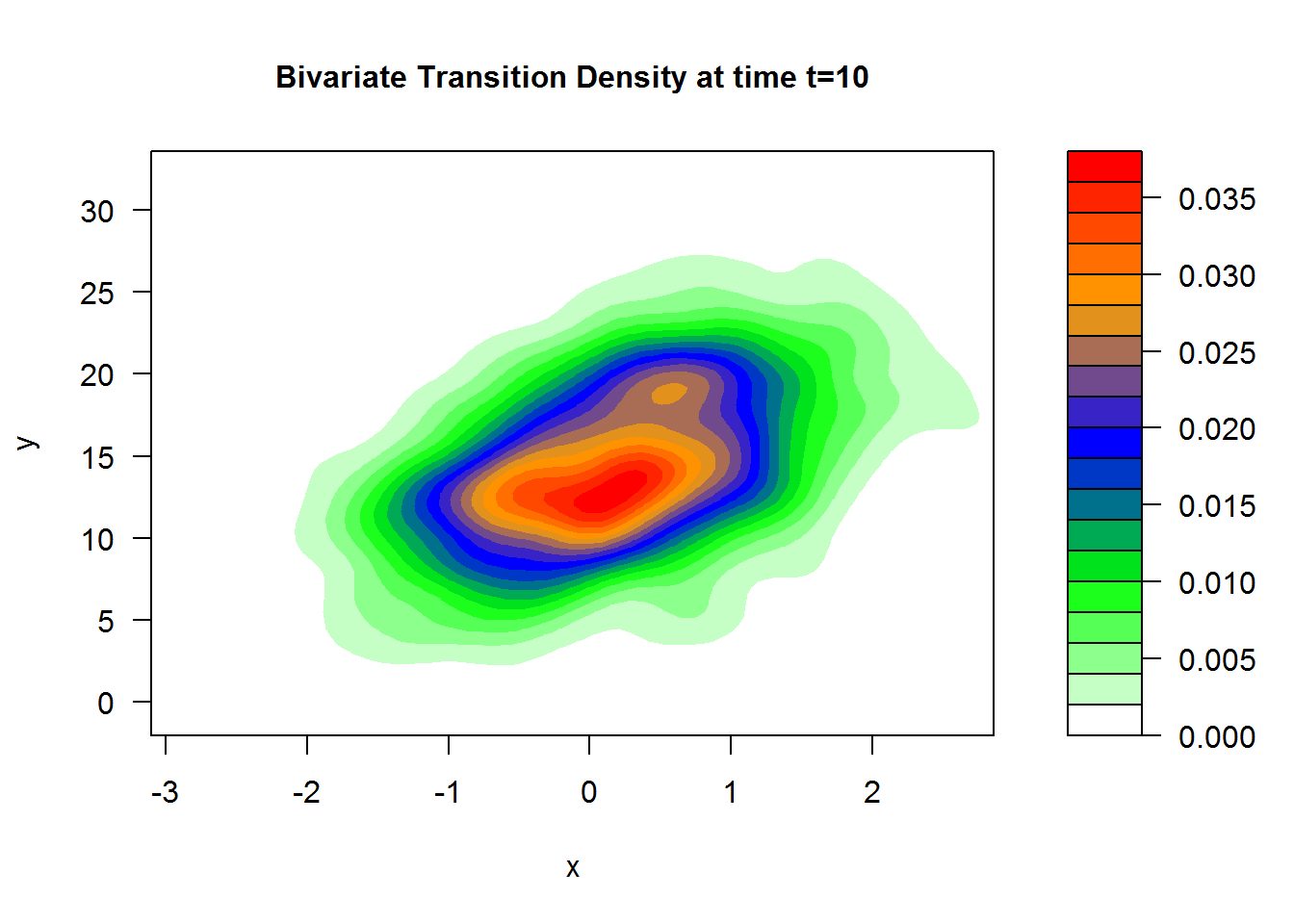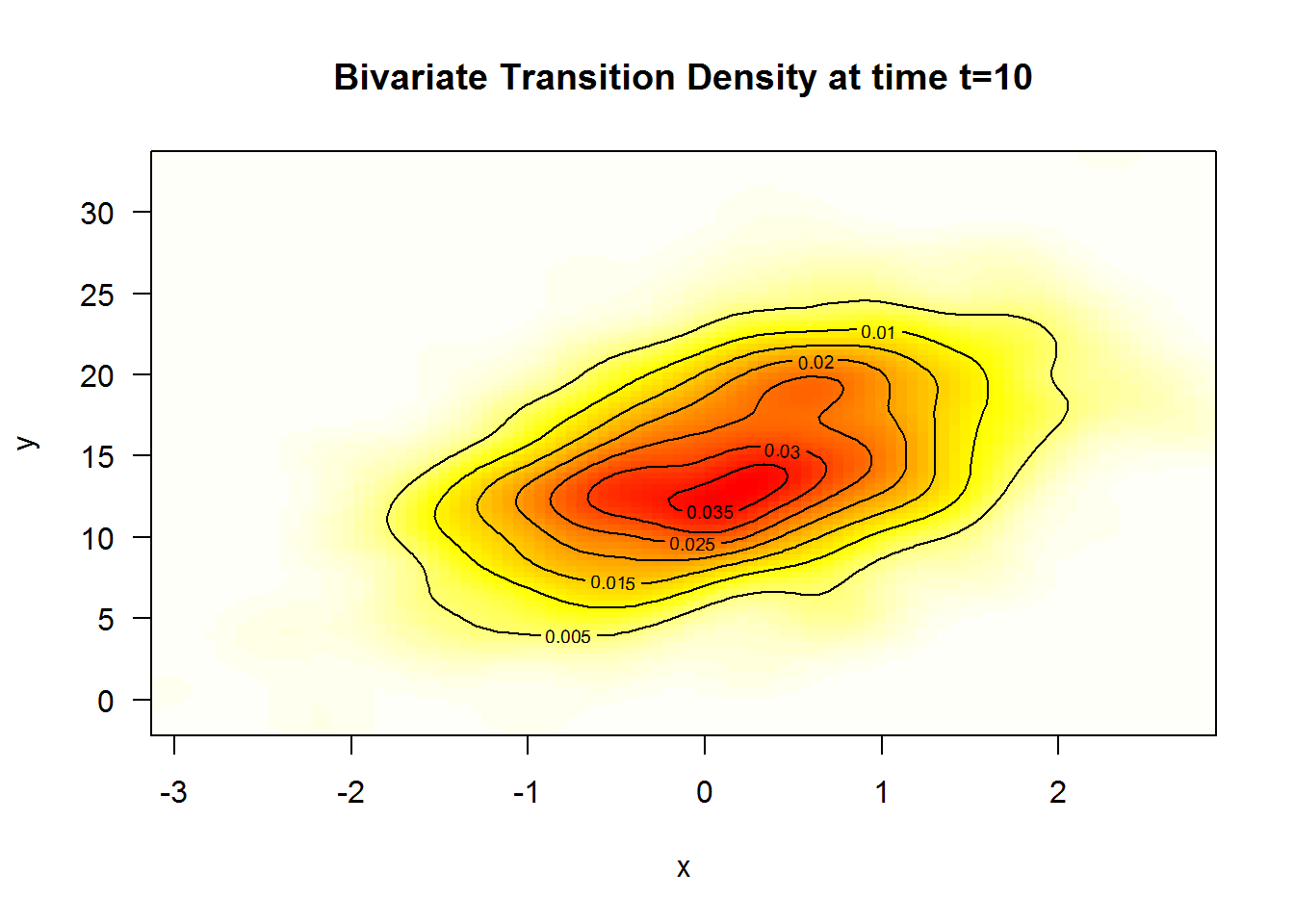A $$3$$D plot of the transition density at $$t=10$$ obtained with: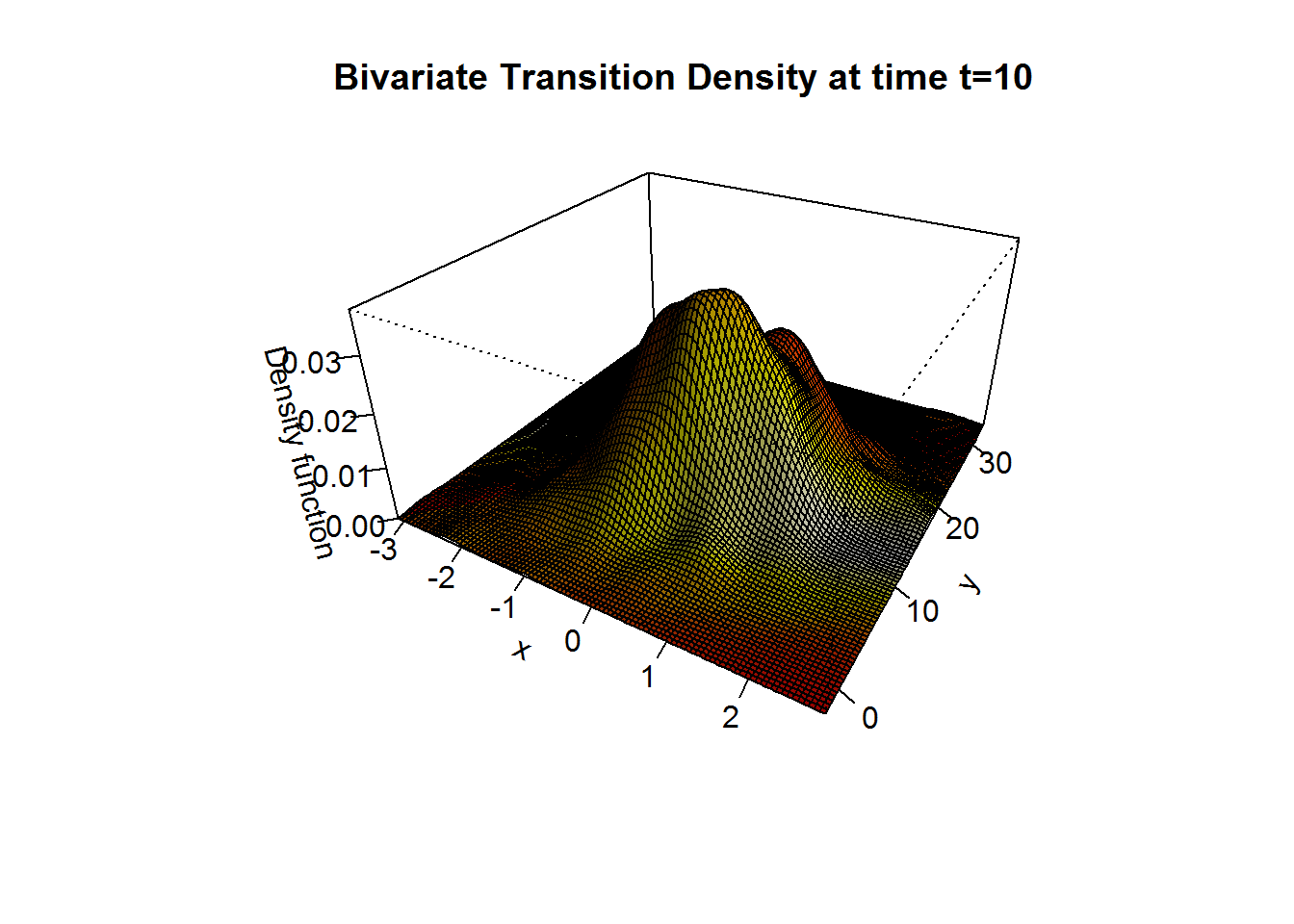We approximate the bivariate transition density over the set transition horizons $$t\in [1,10]$$ by $$\Delta t = 0.005$$ using the code:

The stochastic Van-der-Pol equation

The Van der Pol (1922) equation is an ordinary differential equation that can be derived from the Rayleigh differential equation by differentiating and setting $$\dot{x}=y$$, see Naess and Hegstad (1994); Leung (1995) and for more complex dynamics in Van-der-Pol equation see Jing et al. (2006). It is an equation describing self-sustaining oscillations in which energy is fed into small oscillations and removed from large oscillations. This equation arises in the study of circuits containing vacuum tubes and is given by: $\begin{equation}\label{eq:12} \ddot{X}-\mu (1-X^{2}) \dot{X} + X = 0 \end{equation}$ where $$x$$ is the position coordinate (which is a function of the time $$t$$), and $$\mu$$ is a scalar parameter indicating the nonlinearity and the strength of the damping, to simulate the deterministic equation see Grayling (2014) for more details. Consider stochastic perturbations of the Van-der-Pol equation, and random excitation force of such systems by White noise $$\xi_{t}$$, with delta-type correlation function $$\text{E}(\xi_{t}\xi_{t+h})=2\sigma \delta (h)$$ $\begin{equation}\label{eq:13} \ddot{X}-\mu (1-X^{2}) \dot{X} + X = \xi_{t}, \end{equation}$ where $$\mu > 0$$ . It’s solution cannot be obtained in terms of elementary functions, even in the phase plane. The White noise $$\xi_{t}$$ is formally derivative of the Wiener process $$W_{t}$$. The representation of a system of two first order equations follows the same idea as in the deterministic case by letting $$\dot{x}=y$$, from physical equation we get the above system: $\begin{equation}\label{eq:14} \begin{cases} \dot{X} = Y \\ \dot{Y} = \mu \left(1-X^{2}\right) Y - X + \xi_{t} \end{cases} \end{equation}$ The system can mathematically explain by a Stratonovitch equations: $\begin{equation}\label{eq:15} \begin{cases} dX_{t} = Y_{t} dt \\ dY_{t} = \left(\mu (1-X^{2}_{t}) Y_{t} - X_{t}\right) dt + 2 \sigma \circ dW_{2,t} \end{cases} \end{equation}$

Implemente in R as follows, with integration step size $$\Delta t = 0.01$$ and using stochastic Runge-Kutta methods 1-stage.

Stratonovich Sde 2D:
| dX(t) = Y(t) * dt + 0 o dW1(t)
| dY(t) = (mu * (1 - X(t)^2) * Y(t) - X(t)) * dt + 2 * sigma o dW2(t)
Method:
| Runge-Kutta method with order 1
Summary:
| Size of process   | N  = 10001.
| Number of simulation  | M  = 1.
| Initial values    | (x0,y0) = (0,0).
| Time of process   | t in [0,100].
| Discretization    | Dt = 0.01.

For plotting (back in time) using the command plot, and plot2d in plane the results of the simulation are shown in Figure 8.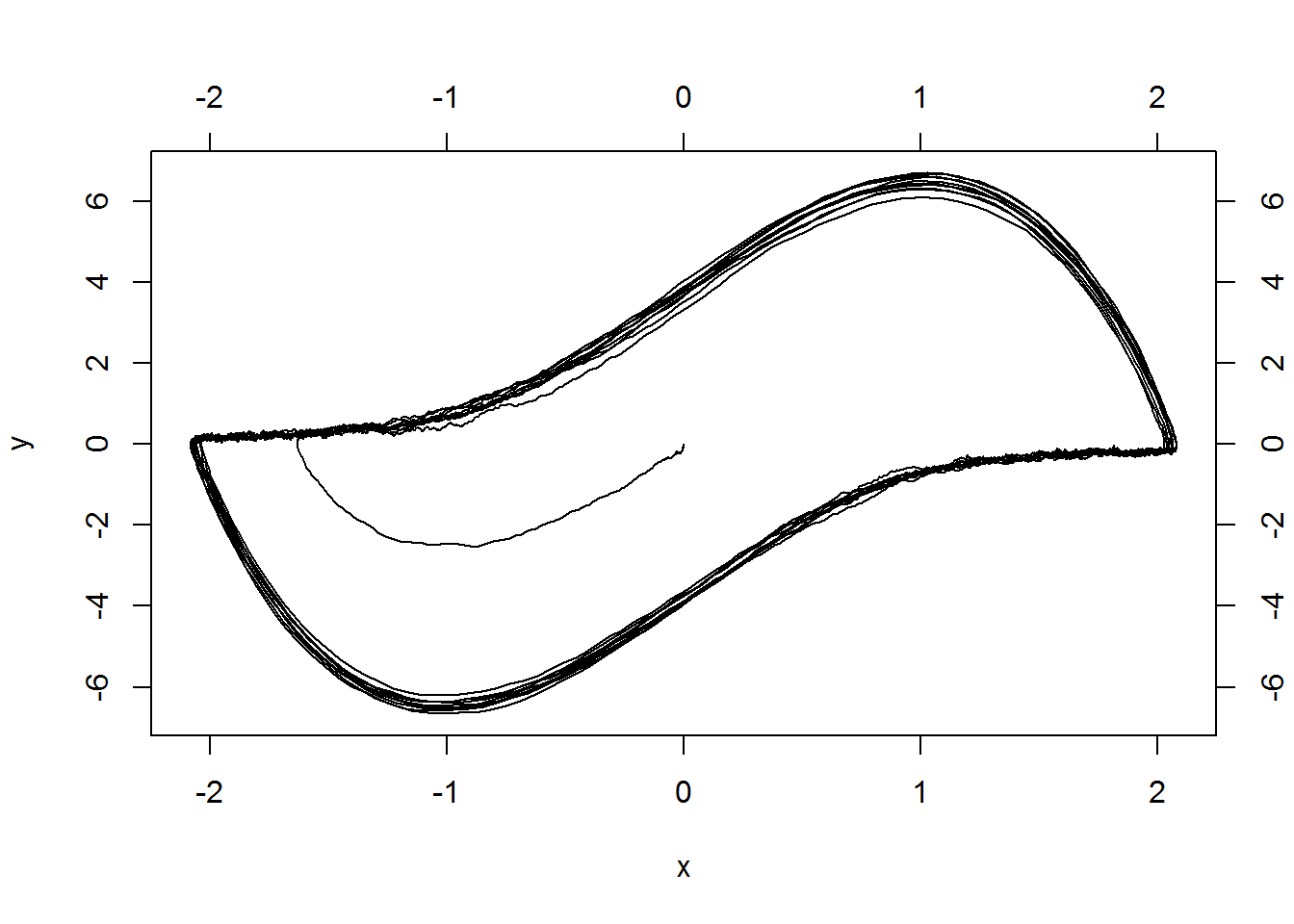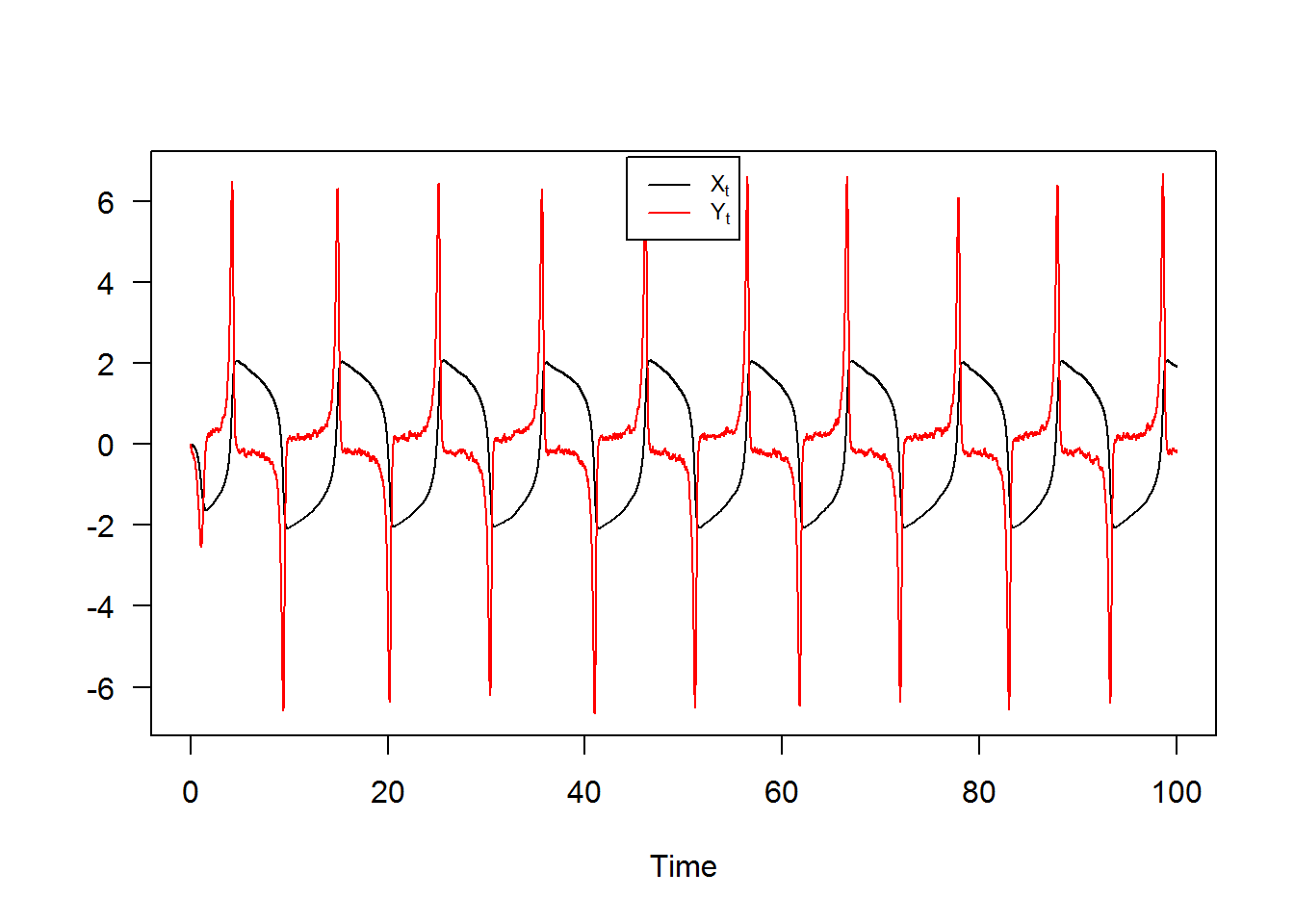snssde3d()

The following $$3$$-dimensional SDE’s with a vector of drift and a diagonal matrix of diffusion coefficients:

It? form: $\begin{equation}\label{eq17} \begin{cases} dX_t = f_{x}(t,X_{t},Y_{t},Z_{t}) dt + g_{x}(t,X_{t},Y_{t},Z_{t}) dW_{1,t}\\ dY_t = f_{y}(t,X_{t},Y_{t},Z_{t}) dt + g_{y}(t,X_{t},Y_{t},Z_{t}) dW_{2,t}\\ dZ_t = f_{z}(t,X_{t},Y_{t},Z_{t}) dt + g_{z}(t,X_{t},Y_{t},Z_{t}) dW_{3,t} \end{cases} \end{equation}$

Stratonovich form: $\begin{equation}\label{eq18} \begin{cases} dX_t = f_{x}(t,X_{t},Y_{t},Z_{t}) dt + g_{x}(t,X_{t},Y_{t},Z_{t}) \circ dW_{1,t}\\ dY_t = f_{y}(t,X_{t},Y_{t},Z_{t}) dt + g_{y}(t,X_{t},Y_{t},Z_{t}) \circ dW_{2,t}\\ dZ_t = f_{z}(t,X_{t},Y_{t},Z_{t}) dt + g_{z}(t,X_{t},Y_{t},Z_{t}) \circ dW_{3,t} \end{cases} \end{equation}$ $$W_{1,t}$$, $$W_{2,t}$$ and $$W_{3,t}$$ is a 3 independent standard Wiener process. To simulate this system using snssde3d() function we need to specify:

• The drift (3d) and diffusion (3d) coefficients as R expressions that depend on the state variables x, y , z and time variable t.
• The number of simulation steps N (default: N=1000).
• The number of the solution trajectories to be simulated by M (default: M=1).
• The initial conditions t0, x0 and end time T (default: t0=0, x0=c(0,0,0) and T=1).
• The integration step size Dt (default: Dt=(T-t0)/N).
• The choice of process types by the argument type="ito" for Ito or type="str" for Stratonovich (default type="ito").
• The numerical method to be used by method (default method="euler").

Basic example

Assume that we want to describe the following SDE’s (3D) in It? form: $\begin{equation}\label{eq0166} \begin{cases} dX_t = 4 (-1-X_{t}) Y_{t} dt + 0.2 dW_{1,t}\\ dY_t = 4 (1-Y_{t}) X_{t} dt + 0.2 dW_{2,t}\\ dZ_t = 4 (1-Z_{t}) Y_{t} dt + 0.2 dW_{3,t} \end{cases} \end{equation}$ We simulate a flow of $$10000$$ trajectories, with integration step size $$\Delta t = 0.001$$.

Itô Sde 3D:
| dX(t) = 4 * (-1 - X(t)) * Y(t) * dt + 0.2 * dW1(t)
| dY(t) = 4 * (1 - Y(t)) * X(t) * dt + 0.2 * dW2(t)
| dZ(t) = 4 * (1 - Z(t)) * Y(t) * dt + 0.2 * dW3(t)
Method:
| Euler scheme with order 0.5
Summary:
| Size of process   | N  = 1001.
| Number of simulation  | M  = 1000.
| Initial values    | (x0,y0,z0) = (2,-2,-2).
| Time of process   | t in [0,1].
| Discretization    | Dt = 0.001.

The following statistical measures (S3 method) for class snssde3d() can be approximated for the $$(X_{t},Y_{t},Z_{t})$$ process at any time $$t$$, for example at=1:

 -0.79771  0.87700  0.79586
 0.0098837 0.1097268 0.0099780
 -0.79993  0.83984  0.80182
 -0.78944  0.76454  0.80311
$x 0% 25% 50% 75% 100% -1.08274 -0.86567 -0.79993 -0.73760 -0.35725$y
0%      25%      50%      75%     100%
0.057898 0.644261 0.839842 1.073442 2.203195

\$z
0%     25%     50%     75%    100%
0.38141 0.73503 0.80182 0.86654 1.07719
 3.3793 3.5112 3.7198
  0.32230  0.54207 -0.52200
 -0.12469  0.37790  0.12557
 -1.082739  0.057898  0.381408
 -0.35725  2.20320  1.07719
 0.00033078 0.04236003 0.00037109
 0.44198 1.20952 0.43782

The summary of the results of mod3d at time $$t=1$$ of class snssde3d() is given by:

Monte-Carlo Statistics for (X(t),Y(t),Z(t)) at time t = 1
X       Y        Z
Mean             -0.79771 0.87700  0.79586
Variance          0.00989 0.10984  0.00999
Median           -0.79993 0.83984  0.80182
Mode             -0.78944 0.76454  0.80311
First quartile   -0.86567 0.64426  0.73503
Third quartile   -0.73760 1.07344  0.86654
Minimum          -1.08274 0.05790  0.38141
Maximum          -0.35725 2.20320  1.07719
Skewness          0.32230 0.54207 -0.52200
Kurtosis          3.37935 3.51125  3.71979
Coef-variation   -0.12469 0.37790  0.12557
3th-order moment  0.00032 0.01973 -0.00052
4th-order moment  0.00033 0.04236  0.00037
5th-order moment  0.00004 0.02313 -0.00006
6th-order moment  0.00002 0.03233  0.00003

For plotting (back in time) using the command plot, and plot3D in space the results of the simulation are shown in Figure 9.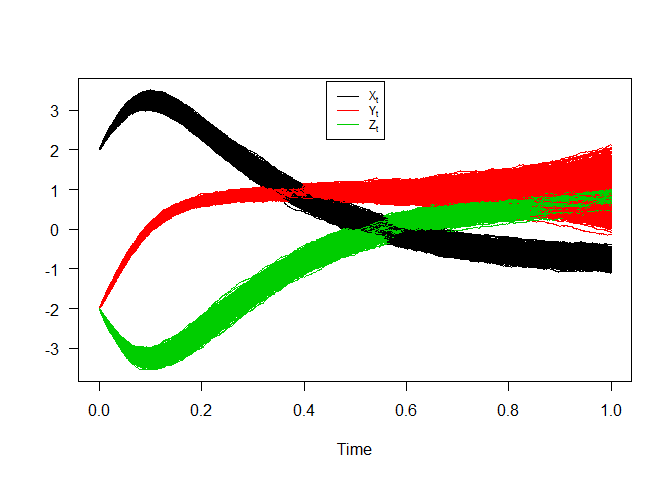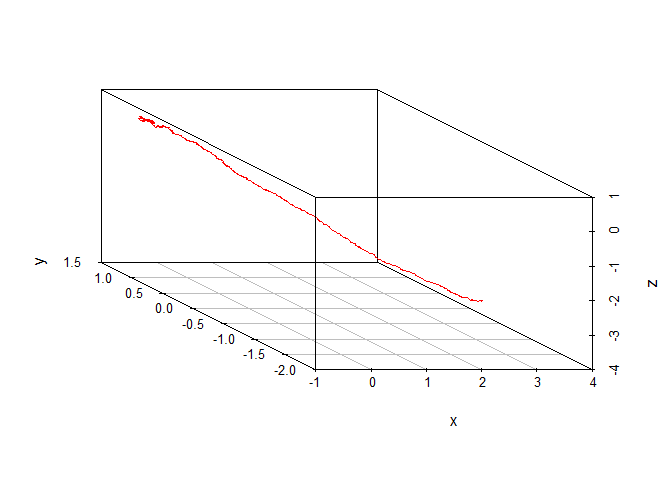3D SDEs

Hence we can just make use of the rsde3d() function to build our random number for $$(X_{t},Y_{t},Z_{t})$$ at time $$t = 1$$.

x       y       z
1  -0.56659 0.40010 0.58340
2  -0.83126 0.54093 0.65433
3  -0.96898 1.30182 0.82791
4  -0.85467 0.91820 0.82323
5  -0.74002 0.85950 0.72057
6  -0.72922 0.84603 0.88307
7  -0.68068 0.56023 0.57196
8  -0.77963 0.62726 0.75782
9  -0.61304 0.54800 0.72629
10 -0.86653 1.26019 0.94123
x                y                z
Min.   :-1.083   Min.   :0.0579   Min.   :0.381
1st Qu.:-0.866   1st Qu.:0.6443   1st Qu.:0.735
Median :-0.800   Median :0.8398   Median :0.802
Mean   :-0.798   Mean   :0.8770   Mean   :0.796
3rd Qu.:-0.738   3rd Qu.:1.0734   3rd Qu.:0.867
Max.   :-0.357   Max.   :2.2032   Max.   :1.077
x         y          z
x  0.0098936 -0.018792 -0.0043937
y -0.0187917  0.109837  0.0200126
z -0.0043937  0.020013  0.0099880

For each SDE type and for each numerical scheme, the marginal density of $$X_t$$, $$Y_t$$ and $$Z_t$$ at time $$t=1$$ are reported using dsde3d() function, see e.g. Figure 10.

Marginal density of X(t-t0)|X(t0)=2 at time t = 1

Data: x (1000 obs.);    Bandwidth 'bw' = 0.02161

x                 f(x)
Min.   :-1.14756   Min.   :0.0002
1st Qu.:-0.93378   1st Qu.:0.0279
Median :-0.72000   Median :0.4314
Mean   :-0.72000   Mean   :1.1683
3rd Qu.:-0.50621   3rd Qu.:2.1636
Max.   :-0.29243   Max.   :4.0682

Marginal density of Y(t-t0)|Y(t0)=-2 at time t = 1

Data: y (1000 obs.);    Bandwidth 'bw' = 0.07241

y                 f(y)
Min.   :-0.15932   Min.   :0.00006
1st Qu.: 0.48561   1st Qu.:0.02870
Median : 1.13055   Median :0.17131
Mean   : 1.13055   Mean   :0.38726
3rd Qu.: 1.77548   3rd Qu.:0.75625
Max.   : 2.42042   Max.   :1.23074

Marginal density of Z(t-t0)|Z(t0)=-2 at time t = 1

Data: z (1000 obs.);    Bandwidth 'bw' = 0.02219

z                f(z)
Min.   :0.31485   Min.   :0.0002
1st Qu.:0.52207   1st Qu.:0.0567
Median :0.72930   Median :0.4489
Mean   :0.72930   Mean   :1.2052
3rd Qu.:0.93653   3rd Qu.:2.3340
Max.   :1.14375   Max.   :4.2142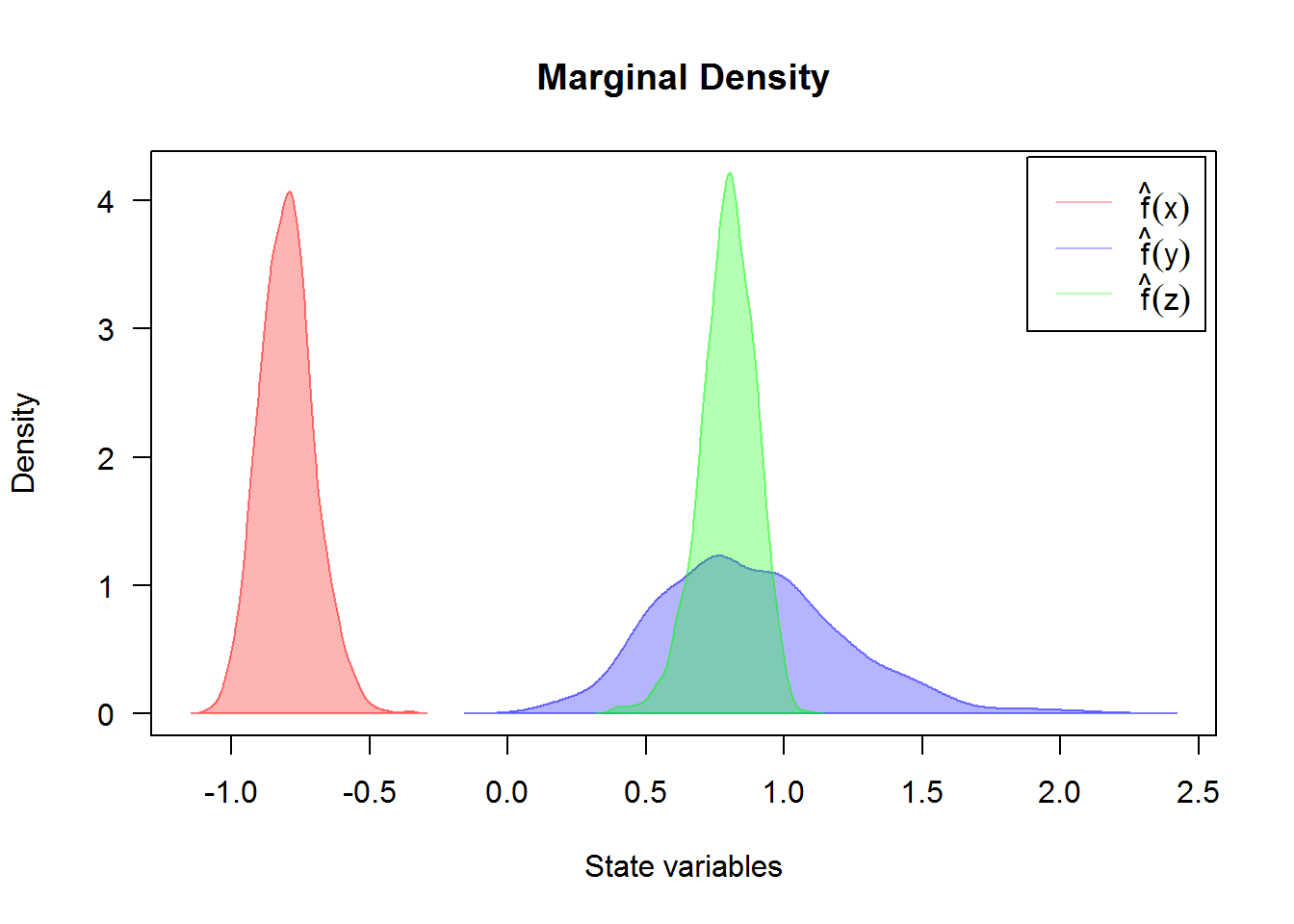For an approximate joint transition density for $$(X_t,Y_t,Z_t)$$ (for more details, see package sm or ks.)

Attractive model for 3D diffusion processes

If we assume that $$U_w( x , y , z , t )$$, $$V_w( x , y , z , t )$$ and $$S_w( x , y , z , t )$$ are neglected and the dispersion coefficient $$D( x , y , z )$$ is constant. A system becomes (see Boukhetala,1996): $\begin{eqnarray}\label{eq19} % \nonumber to remove numbering (before each equation) \begin{cases} dX_t = \left(\frac{-K X_{t}}{\sqrt{X^{2}_{t} + Y^{2}_{t} + Z^{2}_{t}}}\right) dt + \sigma dW_{1,t} \nonumber\\ dY_t = \left(\frac{-K Y_{t}}{\sqrt{X^{2}_{t} + Y^{2}_{t} + Z^{2}_{t}}}\right) dt + \sigma dW_{2,t} \\ dZ_t = \left(\frac{-K Z_{t}}{\sqrt{X^{2}_{t} + Y^{2}_{t} + Z^{2}_{t}}}\right) dt + \sigma dW_{3,t} \nonumber \end{cases} \end{eqnarray}$ with initial conditions $$(X_{0},Y_{0},Z_{0})=(1,1,1)$$, by specifying the drift and diffusion coefficients of three processes $$X_{t}$$, $$Y_{t}$$ and $$Z_{t}$$ as R expressions which depends on the three state variables (x,y,z) and time variable t, with integration step size Dt=0.0001.

Itô Sde 3D:
| dX(t) = (-K * X(t)/sqrt(X(t)^2 + Y(t)^2 + Z(t)^2)) * dt + sigma * dW1(t)
| dY(t) = (-K * Y(t)/sqrt(X(t)^2 + Y(t)^2 + Z(t)^2)) * dt + sigma * dW2(t)
| dZ(t) = (-K * Z(t)/sqrt(X(t)^2 + Y(t)^2 + Z(t)^2)) * dt + sigma * dW3(t)
Method:
| Euler scheme with order 0.5
Summary:
| Size of process   | N  = 10001.
| Number of simulation  | M  = 1.
| Initial values    | (x0,y0,z0) = (1,1,1).
| Time of process   | t in [0,1].
| Discretization    | Dt = 0.0001.

The results of simulation are shown: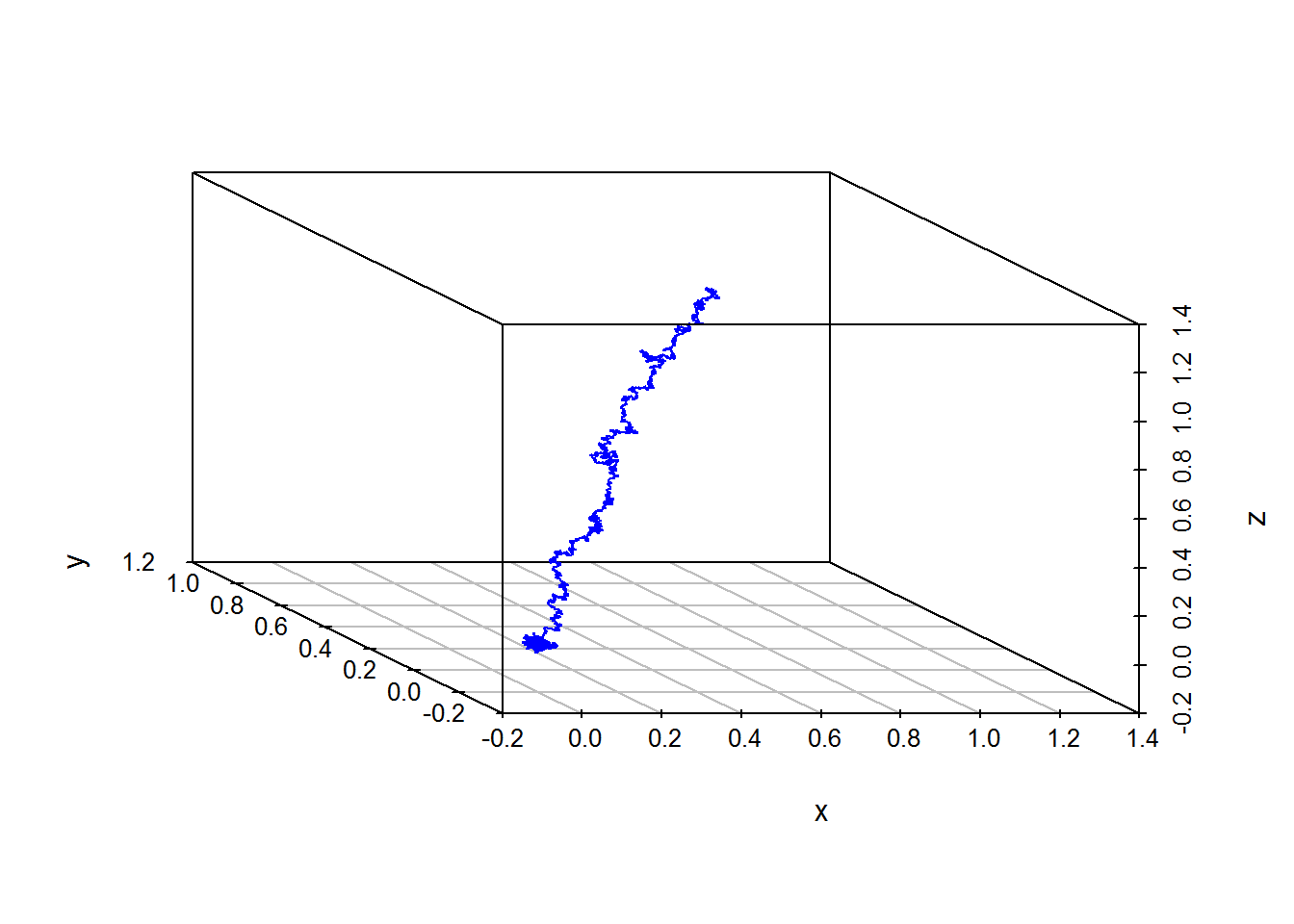Transformation of an SDE one-dimensional

Next is an example of one-dimensional SDE driven by three independent Brownian motions ($$W_{1,t}$$,$$W_{2,t}$$,$$W_{3,t}$$), as follows: $\begin{equation}\label{eq20} dX_{t} = \mu W_{1,t} dt + \sigma W_{2,t} \circ dW_{3,t} \end{equation}$ To simulate the solution of the process $$X_t$$, we make a transformation to a system of three equations as follows: $\begin{eqnarray}\label{eq21} \begin{cases} % \nonumber to remove numbering (before each equation) dX_t = \mu Y_{t} dt + \sigma Z_{t} \circ dW_{3,t} \nonumber\\ dY_t = dW_{1,t} \\ dZ_t = dW_{2,t} \nonumber \end{cases} \end{eqnarray}$ run by calling the function snssde3d() to produce a simulation of the solution, with $$\mu = 1$$ and $$\sigma = 1$$.

Stratonovich Sde 3D:
| dX(t) = Y(t) * dt + Z(t) o dW1(t)
| dY(t) = 0 * dt + 1 o dW2(t)
| dZ(t) = 0 * dt + 1 o dW3(t)
Method:
| Euler scheme with order 0.5
Summary:
| Size of process   | N  = 1001.
| Number of simulation  | M  = 1000.
| Initial values    | (x0,y0,z0) = (0,0,0).
| Time of process   | t in [0,1].
| Discretization    | Dt = 0.001.

Monte-Carlo Statistics for (X(t),Y(t),Z(t)) at time t = 1
X          Y         Z
Mean              0.02050   -0.00735   0.00410
Variance          0.76290    1.02520   0.92697
Median           -0.00513    0.00347  -0.00932
Mode              0.08659   -0.10735  -0.07669
First quartile   -0.50274   -0.69009  -0.63180
Third quartile    0.52399    0.69699   0.66827
Minimum          -2.80265   -3.26565  -3.90726
Maximum           5.99953    3.72179   3.17907
Skewness          0.46773   -0.03733  -0.12839
Kurtosis          5.74922    3.15054   3.41486
Coef-variation   42.61668 -137.73620 234.60715
3th-order moment  0.31167   -0.03875  -0.11459
4th-order moment  3.34617    3.31130   2.93428
5th-order moment  8.66966    0.11897  -1.59067
6th-order moment 56.80855   18.73499  18.05038

the following code produces the result in Figure 12.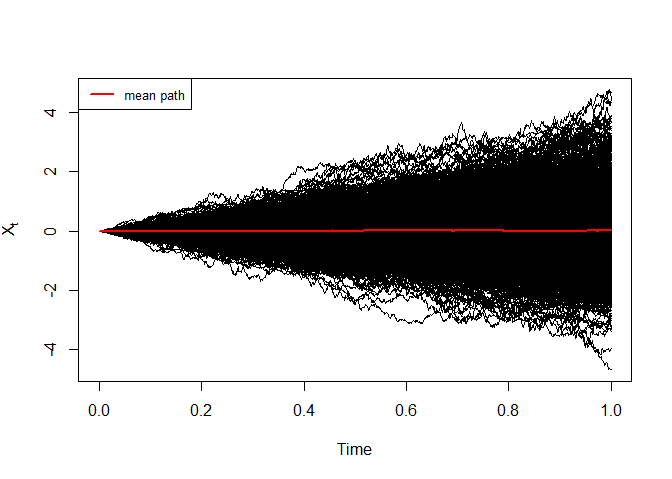Simulation of $$X_t$$

The histogram and kernel density of $$X_t$$ at time $$t=1$$ are reported using dsde3d() function, see e.g. Figure 13.

Call:
density.default(x = x, na.rm = TRUE)

Data: x (1000 obs.);    Bandwidth 'bw' = 0.1732

x                y
Min.   :-3.322   Min.   :0.00000
1st Qu.:-0.862   1st Qu.:0.00058
Median : 1.598   Median :0.01768
Mean   : 1.598   Mean   :0.10151
3rd Qu.: 4.059   3rd Qu.:0.14044
Max.   : 6.519   Max.   :0.50564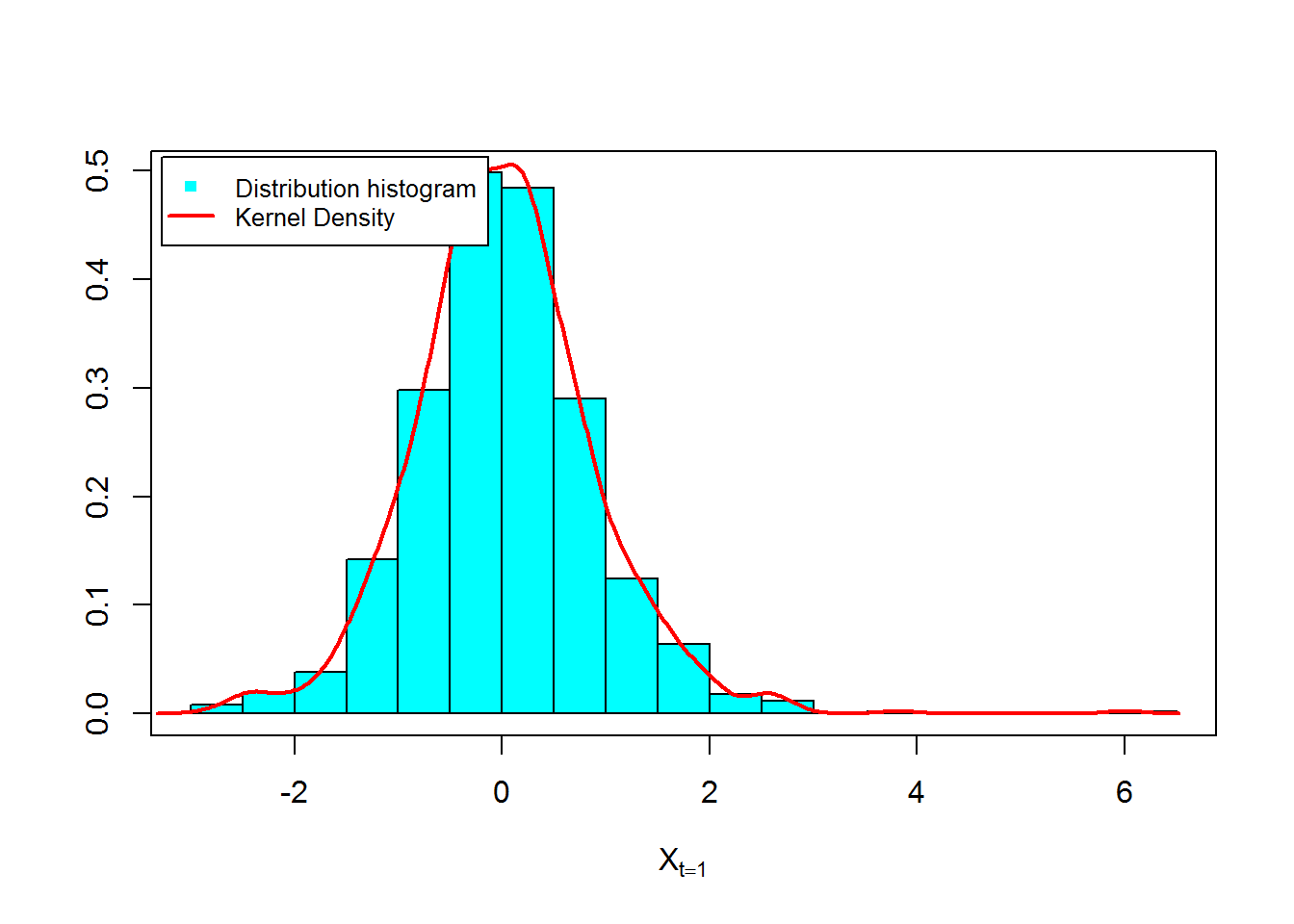Figure 14 and 15, show approximation results for $$m_{1}(t)= \text{E}(X_{t})$$, $$S_{1}(t)=\text{V}(X_{t})$$ and $$C(s,t)=\text{Cov}(X_{s},X_{t})$$: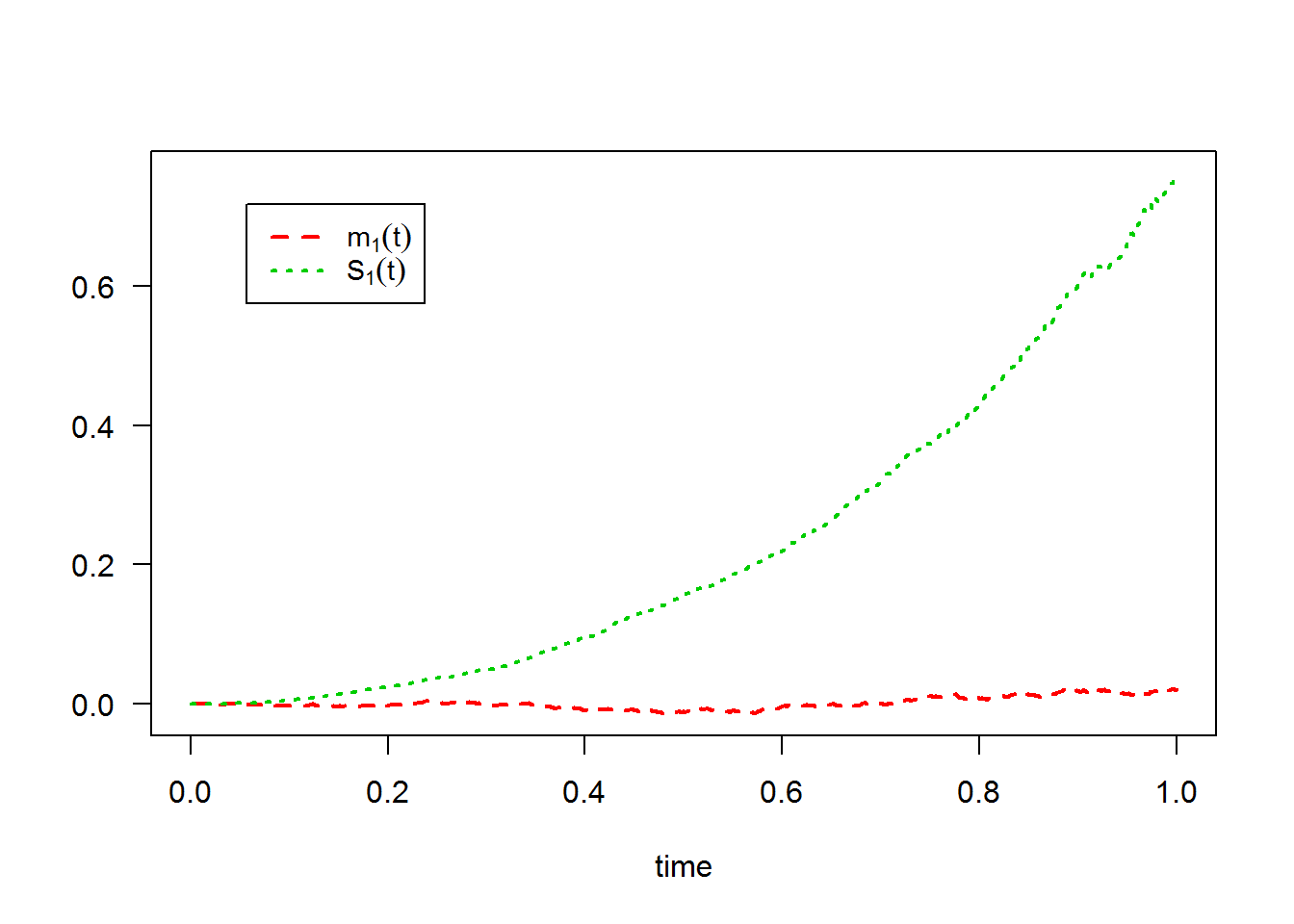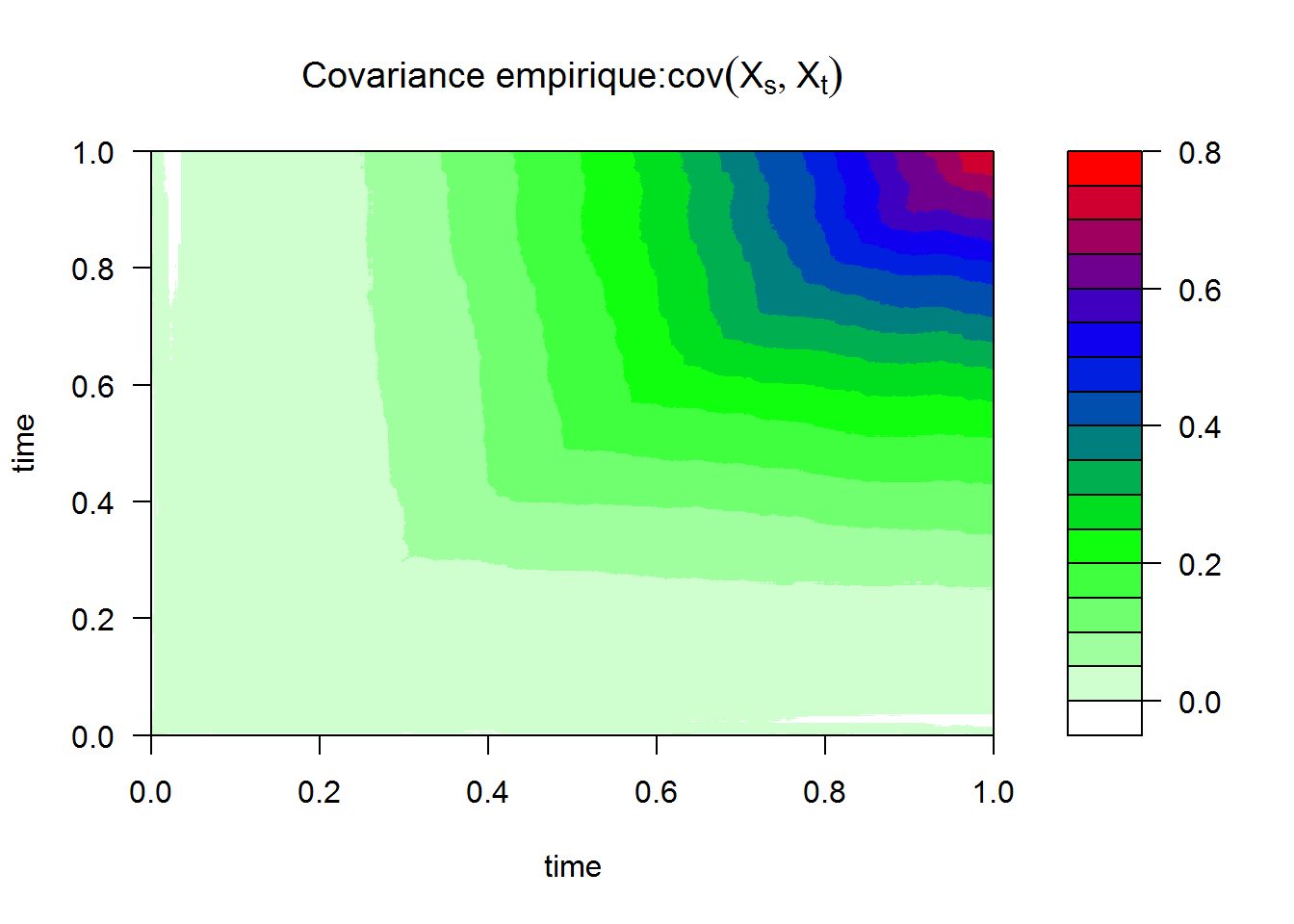3. The equivalently of $$X_{t}^{\text{mod1}}$$ the following Stratonovich SDE: $$dX_{t} = \theta X_{t} \circ dW_{t}$$.